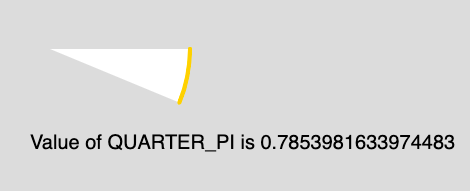GeeksforGeeks App
Open AppBrowser
Continue

## Related Articles

The QUARTER_PI is a mathematical constant with the value 0.7853982. It is one quarter the ratio of the circumference of a circle to its diameter.

Syntax:

`QUARTER_PI`

Below program illustrates the QUARTER_PI constant in p5.js:

Example: This example describes the QUARTER_PI constant.

 `function` `setup() {`` ` `    ``// Create Canvas of size 880*300``    ``createCanvas(880, 300);``}`` ` `function` `draw() {``     ` `    ``// Set the background Color``    ``background(220);``     ` `    ``// Set the stroke color``    ``stroke(255, 204, 0);``     ` `    ``// Set the stroke weight``    ``strokeWeight(4);``     ` `    ``// Use of constant QUARTER_PI``    ``arc(50, 50, 280, 280, 0, QUARTER_PI/2); ``     ` `    ``noStroke();``     ` `    ``// Set font size``    ``textSize(20);``     ` `    ``// Display result``    ``text(``"Value of QUARTER_PI is "` `            ``+ QUARTER_PI, 30, 150);``}`

Output:My Personal Notes arrow_drop_up
Related Tutorials